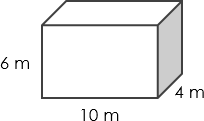# Volume Calculator

This is a cube volume calculator (cuboid volume calculator) that helps you calculate the volume of a cube provided that you know its length, width, and height. Use this online calculator for a quick calculation.

## How to calculate the volume of a cube?

To find out the volume of a cube, we need to know its length, width, and height. The volume is found using the formula:

Volume = `Length × Width × Height` (Symbolic form: `v = l * w * h` or `v=lwh`)

It doesn’t really matter which side of a cube is length, width or height, so long as you multiply all three together.

## Volume calculation example

Find the volume of the cube below:The volume is: 10 m x 4 m x 6 m = 240 m3

## How to use this volume calculator?

To use our volume calculation tool, walk through the steps below:

1. Enter the width, length, and height of the cube. Again, it doesn’t matter which side of a cube is length, width or height.
2. Check the result.

#### This calculator is created with

the best online spreadsheet editor with excellent formula and editing capability.

For more calculators for finance, mathematics health, unit converters and more check out our calculators collection.